## ROC curves and AUC:

There are reference answers on-line here here, here and here.

DEFINITIONS:

$$\text{Sensitivity or TP Rate} = \frac{TP}{TP\, +\, FN}$$

$$\text{Specificity} = \frac{TN}{TN\, +\, FP}$$

$$\text{FP Rate or Fall-out} = \frac{FP}{TN\, +\, FP} = 1 - \text{Specificity}$$

An accuracy of 100% means that the measured values are exactly the same as the given values:

$$\text{Accuracy} = \frac{TP\, +\, TN}{TP\, +\, TN\, +\, FP\, +\, FN}$$

$$\text{Precision or PPV} = \frac{TP}{TP\, +\, FP}$$

$$\text{NPV} = \frac{TN}{TN\, +\, FN}$$

Utilizing the PSA antigen data from here, where fpsa stands for “free PSA” and tpsa stands for “total PSA”. There are repeated measures in time t at different age-s. The outcome is d and is coded as 0 or 1:

library(gsubfn)
library(sqldf)
library(tcltk)
library(pROC)
library(ROCR)
library(Epi)
library(gsheet)

The following is the dataset from a an age-matched case-control study: each diagnosed case was assigned a control matched to case by date of birth. There are 70 non diagnosed and 71 diagnosed:

data = read.csv(text = gsheet2text('https://docs.google.com/spreadsheets/d/1ba1IYjX79EEmgBmsEzZgNs6Joe4v-SECWZUhbfhewhc/edit?usp=sharing', format ='csv'))
## No encoding supplied: defaulting to UTF-8.
data = sqldf("select id, d, min(t), fpsa, tpsa, age from 'data'\n group by id")
## Warning: Quoted identifiers should have class SQL, use DBI::SQL() if the
## caller performs the quoting.
data$age_group <- cut(data$age, breaks = c(45,55,65,75), labels = c("(45,55]","(55,65]","(65,75]"))
colnames(data) = "outcome"
head(data)
##   id outcome min(t)  fpsa  tpsa    age age_group
## 1  1       1 -4.482 3.525 14.82 67.581   (65,75]
## 2  2       1 -4.498 1.104  5.54 70.166   (65,75]
## 3  3       0 -3.381 0.226  0.94 55.028   (55,65]
## 4  4       1 -4.619 0.583  9.30 57.424   (55,65]
## 5  5       0 -4.005 0.377  1.71 62.732   (55,65]
## 6  6       1 -0.337 0.150  0.58 58.360   (55,65]

Generating ROC curves with pROC:

# With total PSA:

r = roc(data$outcome, data$tpsa)
plot.roc(r, print.auc=TRUE, auc.polygon=TRUE, auc.polygon.col=rgb(.3,0,.8,0.2), print.thres=TRUE)
plot.roc(smooth(r), add=TRUE, col=2)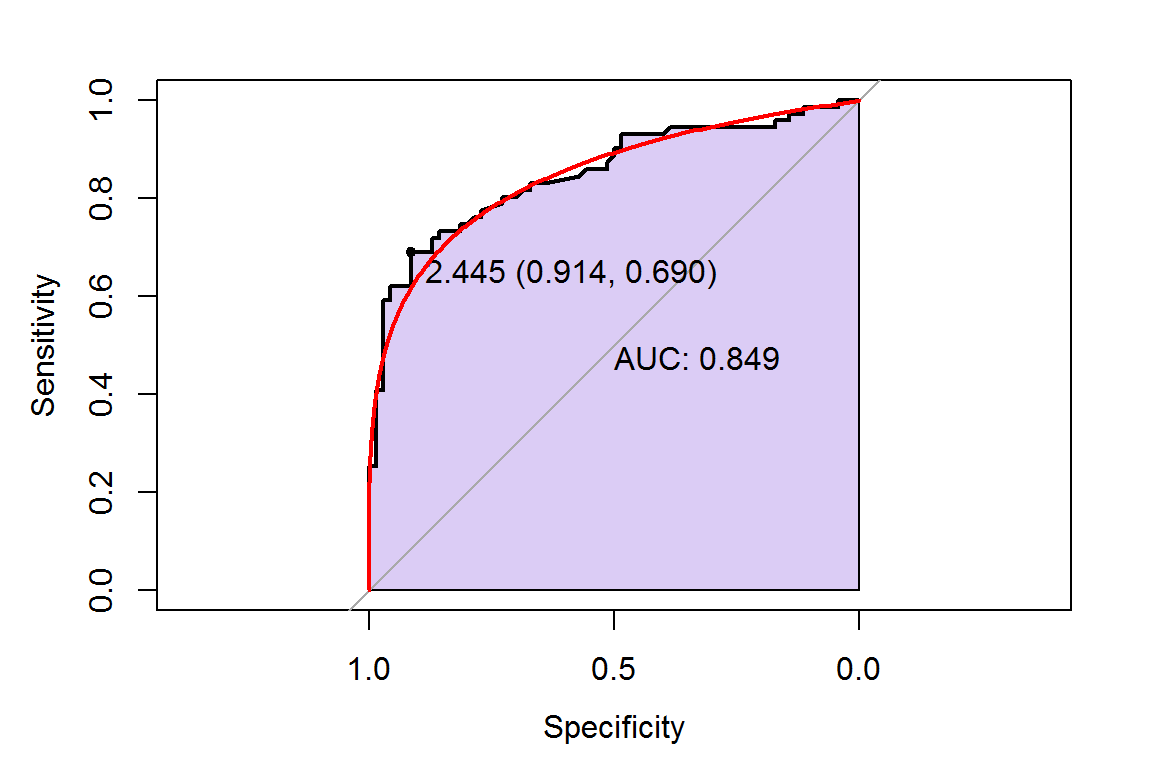Notice that the thresholds used to construct the ROC plot, together with the calculated sensitivities and specificities are stored in roc:

rock = pROC::roc(data$outcome, data$tpsa)
str(rock)
## List of 15
##  $percent : logi FALSE ##$ sensitivities     : num [1:120] 1 1 1 0.986 0.986 ...
##  $specificities : num [1:120] 0 0.0286 0.0429 0.0429 0.0571 ... ##$ thresholds        : num [1:120] -Inf 0.3 0.385 0.445 0.455 ...
##  $direction : chr "<" ##$ cases             : num [1:71] 14.82 5.54 9.3 0.58 13.61 ...
##  $controls : num [1:70] 0.94 1.71 0.96 0.27 1.04 1.93 1.5 0.92 4.47 0.45 ... ##$ fun.sesp          :function (thresholds, controls, cases, direction)
##  $auc :Classes 'auc', 'numeric' atomic [1:1] 0.849 ## .. ..- attr(*, "partial.auc")= logi FALSE ## .. ..- attr(*, "percent")= logi FALSE ## .. ..- attr(*, "roc")=List of 8 ## .. .. ..$ percent      : logi FALSE
##   .. .. ..$sensitivities: num [1:120] 1 1 1 0.986 0.986 ... ## .. .. ..$ specificities: num [1:120] 0 0.0286 0.0429 0.0429 0.0571 ...
##   .. .. ..$thresholds : num [1:120] -Inf 0.3 0.385 0.445 0.455 ... ## .. .. ..$ direction    : chr "<"
##   .. .. ..$cases : num [1:71] 14.82 5.54 9.3 0.58 13.61 ... ## .. .. ..$ controls     : num [1:70] 0.94 1.71 0.96 0.27 1.04 1.93 1.5 0.92 4.47 0.45 ...
##   .. .. ..$fun.sesp :function (thresholds, controls, cases, direction) ## .. .. ..- attr(*, "class")= chr "roc" ##$ call              : language roc.default(response = data$outcome, predictor = data$tpsa)
##  $original.predictor: num [1:141] 14.82 5.54 0.94 9.3 1.71 ... ##$ original.response : int [1:141] 1 1 0 1 0 1 0 1 0 1 ...
##  $predictor : num [1:141] 14.82 5.54 0.94 9.3 1.71 ... ##$ response          : int [1:141] 1 1 0 1 0 1 0 1 0 1 ...
##  $levels : chr [1:2] "0" "1" ## - attr(*, "class")= chr "roc" # Let's take a peek under the hood: roc_scaffolding = data.frame(rock$thresholds, rock$sensitivities, rock$specificities)
head(roc_scaffolding)
##   rock.thresholds rock.sensitivities rock.specificities
## 1            -Inf          1.0000000         0.00000000
## 2           0.300          1.0000000         0.02857143
## 3           0.385          1.0000000         0.04285714
## 4           0.445          0.9859155         0.04285714
## 5           0.455          0.9859155         0.05714286
## 6           0.480          0.9859155         0.07142857

In addition to the PSA we can stratify by patient’s age:

# With age groups:

plot.roc(x = data$outcome[data$age_gr == "(45,55]"],
predictor = data$tpsa[data$age_gr == "(45,55]"],
print.auc = TRUE, col = "green", print.auc.col = "green",
print.auc.y = 0.97, print.auc.x = 0.5)

plot.roc(x = data$outcome[data$age_gr == "(55,65]"],
predictor = data$tpsa[data$age_gr == "(55,65]"],
print.auc = TRUE, col = "blue", print.auc.col = "blue", add = TRUE,
print.auc.y = 0.82, print.auc.x = 0.7)

plot.roc(x = data$outcome[data$age_gr == "(65,75]"],
predictor = data$tpsa[data$age_gr == "(65,75]"],
print.auc = TRUE, col = "red", add = TRUE, print.auc.col = "red",
print.auc.y = 0.7, print.auc.x = 0.8)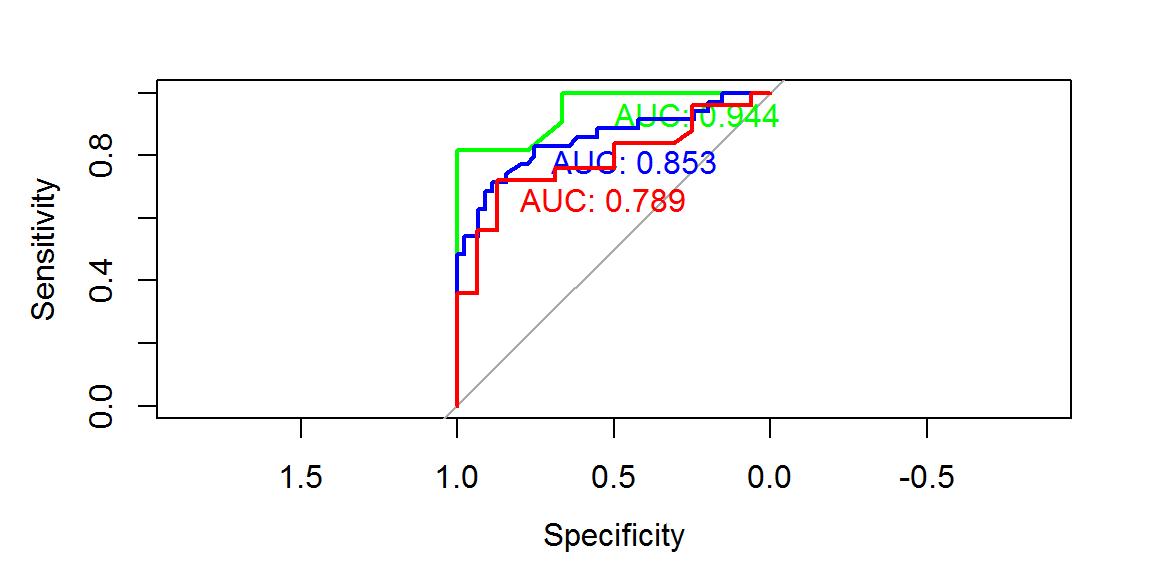And with ROCR:

# With ROCR:
pred <- prediction(data$tpsa, data$outcome)
perf <- performance(pred, "tpr", "fpr")
# performance metrics TPR: True Positive Ratio FPR: False Positive Ratio
plot(perf, col = "green")
abline(0, 1, col = "grey")
auc <- performance(pred, "auc")
legend("bottomright", paste(round(as.numeric(auc@y.values), digits = 2)), col = c("green"), pch = c(3))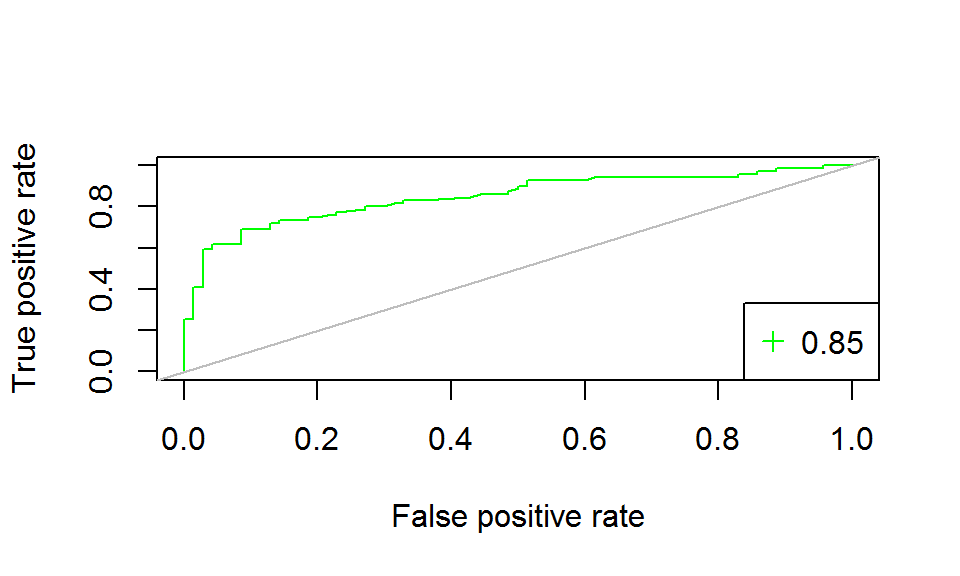Alternatively, the Epi package can be used. In this case we are dealing with predicting the clinical outcome in patients with subarachnoid hemorrage.

data(aSAH)
head(aSAH)
##    gos6 outcome gender age wfns s100b  ndka
## 29    5    Good Female  42    1  0.13  3.01
## 30    5    Good Female  37    1  0.14  8.54
## 31    5    Good Female  42    1  0.10  8.09
## 32    5    Good Female  27    1  0.04 10.42
## 33    1    Poor Female  42    3  0.13 17.40
## 34    1    Poor   Male  48    2  0.10 12.75
rock = ROC(form = outcome ~ s100b, data=aSAH, plot = "ROC", MX = T)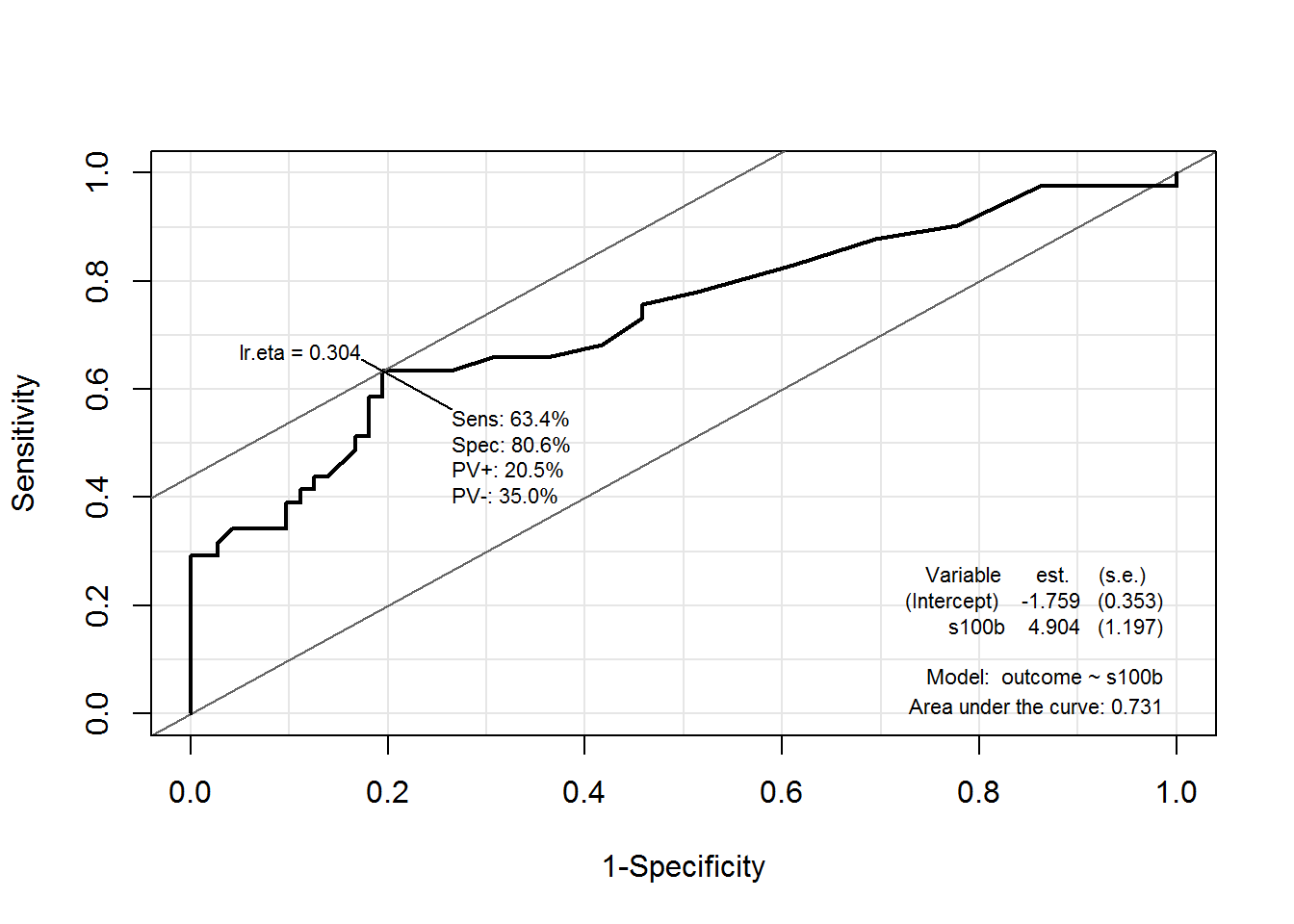Notice the values in the $$18$$-th row of the ROC$res printout - they are the values printed on plot above as lr.eta values: rock$res[18,]
##                       sens      spec       pvp  pvn   lr.eta
## 0.30426295405785 0.6341463 0.8055556 0.2054795 0.35 0.304263

The maximized sum threshold or MST or the probability of the outcome is the lr.eta - in other words, the sigmoid function:

$\Large \mathbb{E}[Y_i|X_i]=\frac{1}{1 + e^{-(\beta_0+\beta_1\times\text{s100b})}}$

rc <- ROC(form = outcome ~ s100b, data=aSAH, plot="sp") # Saving the ROC for later
ROC(form = outcome ~ s100b, data=aSAH, plot="sp" ) # Getting the ROC plot
# The following lines just literally color the black lines just printed:
lines(rc$res$lr, rc$res$spec, lwd = 2, type="s", col="red") #specificity
lines(rc$res$lr, rc$res$sens, lwd = 2, type="s", col="green") # sensitivity
lines(rc$res$lr, rc$res$pvp,  lwd = 2, type="s", col="orange") # pvp
lines(rc$res$lr, rc$res$pvn,  lwd = 2, type="s", col="magenta") # pvn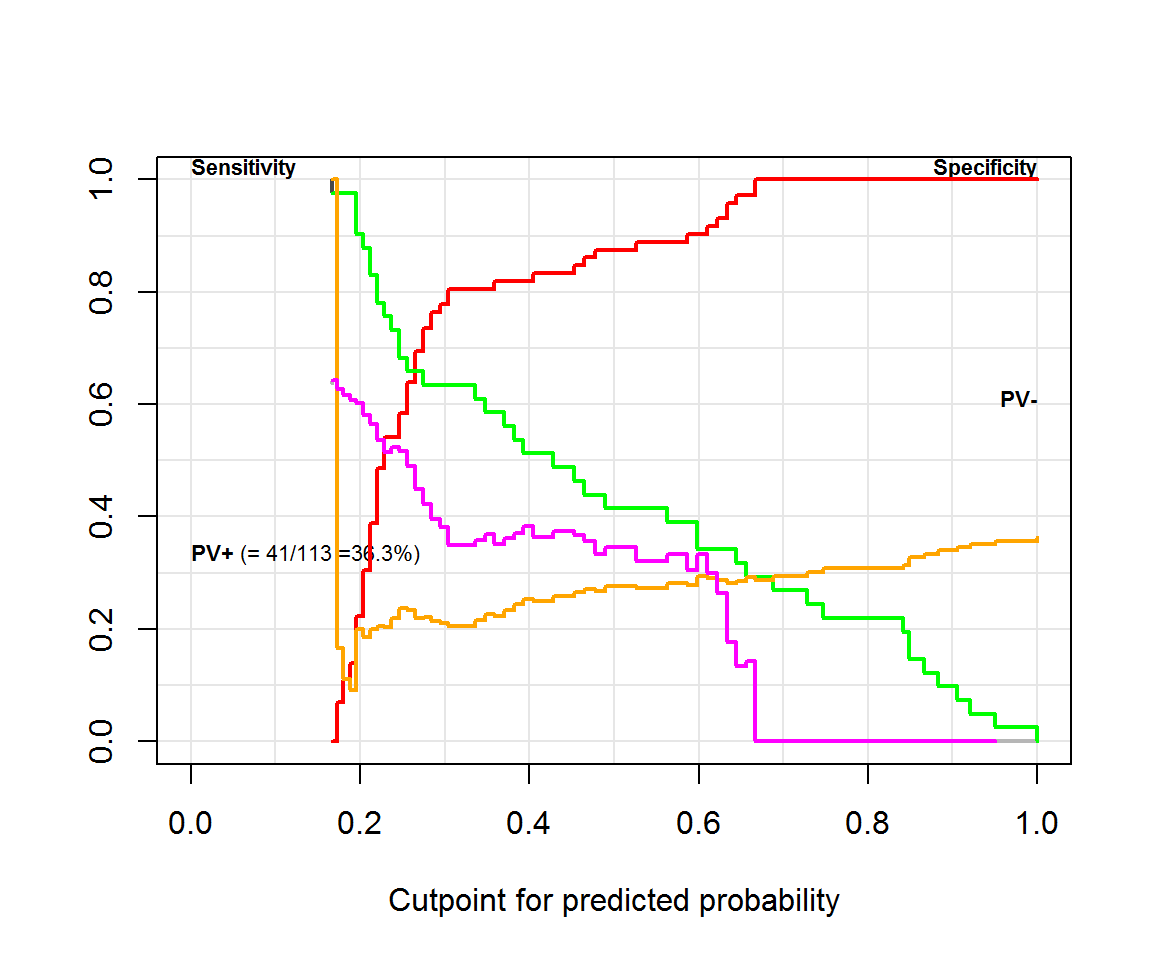The maximal combination of sensitivity and specificity is given by:

## optimal combination of sensitivity and specificity:

which.max(rowSums(rc$res[, c("sens", "spec")])) # Again the same value from row 18! ## 0.30426295405785 ## 18 This is not the way a cut-off value is selected to report the result of a test as positive in clinical practice. Utilities (loss/cost) are needed to solve for the optimum cutoff on predicted risk." See comments here. Given that the parameters of the logistic regression are… rc$lr
##
## Call:  glm(formula = form, family = binomial, data = data)
##
## Coefficients:
## (Intercept)        s100b
##      -1.759        4.904
##
## Degrees of Freedom: 112 Total (i.e. Null);  111 Residual
## Null Deviance:       148
## Residual Deviance: 123.6     AIC: 127.6

this “optimal sensitivity/specificity” value in row 18 corresponds to:

$\large 0.30426295405785 =\frac{1}{1+e^{-(-1.759+4.904\times \text{s100b})}}$

and

$\large \text{s100b}= 0.1900327$

A plausible value of s100b:

summary(aSAH$s100b) # Remember that not all values are used as cutoff points. ## Min. 1st Qu. Median Mean 3rd Qu. Max. ## 0.030 0.090 0.140 0.247 0.330 2.070 that will optimize sensitivity and specificity. If we wanted to get the cutoff points, we’d have to use sort(unique(aSAH)). Let’s see the value in position $$18$$ (points = c("Inf",sort(unique(aSAH$s100b))))
##  "0.19"

#### MANUAL REPRODUCTION:

data("aSAH")
(data <- aSAH[1:5,]) # We take only the first x rows - for instance 5
##    gos6 outcome gender age wfns s100b  ndka
## 29    5    Good Female  42    1  0.13  3.01
## 30    5    Good Female  37    1  0.14  8.54
## 31    5    Good Female  42    1  0.10  8.09
## 32    5    Good Female  27    1  0.04 10.42
## 33    1    Poor Female  42    3  0.13 17.40
contrasts(data$outcome) ## Poor ## Good 0 ## Poor 1 fit <- glm(outcome ~ s100b, data, family = "binomial") # This is the logistic regression that we will use as a predictor. ODDS RATIOS: exp(coef(fit)) ## (Intercept) s100b ## 1.571381e-03 1.690125e+18 exp(confint(fit)) ## 2.5 % 97.5 % ## (Intercept) 1.499772e-22 1.435188e+01 ## s100b 1.165035e-16 1.174063e+160 PROBABILITIES: PROBABILITIES given again by the sigmoid function: $\Large \color{green}{\text{p(Y = 1)}} = \frac{\color{blue}{\text{odds(Y=1)}}}{1\,+\,\color{blue}{\text{odds(Y=1)}}}=\frac{e^{\,\beta_0 + \beta_1 x_1 + \cdots + \beta_p x_p}}{1 \,+\,e^{\,\beta_0 + \beta_1 x_1 + \cdots + \beta_p x_p}}=\frac{1}{1 \,+\,e^{-(\,\beta_0 + \beta_1 x_1 + \cdots + \beta_p x_p)}}$ In a glm() (logistic regression) model, predict() simply gives you the LINEAR EQUATION ($$\beta_0 + \beta_1 x_1 + \cdots + \beta_p x_p$$). probabilities = exp(predict(fit)) / ( 1 + exp(predict(fit)) )  These are the predicted probabilities (not the odds or the results of the logistic regression) for each value of s100b in the dataset. Let’s get the thresholds from the pROC package: (threshold = roc(data$outcome, data$s100b)$thresholds) 
##   -Inf 0.070 0.115 0.135   Inf

Here we have them… Compare them to s100b… Parallel, but not quite:

sort(data$s100b) ##  0.04 0.10 0.13 0.13 0.14 Let’s add the predicted probabilities: data$probabilities <- probabilities

# and order the dataset just to take a peek at what it looks like...

(data[with(data, order(s100b)), ])
##    gos6 outcome gender age wfns s100b  ndka probabilities
## 32    5    Good Female  27    1  0.04 10.42   0.008351337
## 31    5    Good Female  42    1  0.10  8.09   0.094604465
## 29    5    Good Female  42    1  0.13  3.01   0.269034388
## 33    1    Poor Female  42    3  0.13 17.40   0.269034388
## 30    5    Good Female  37    1  0.14  8.54   0.358975423
# Now let's what happens with different cutoff points...

cut <- rep(0, length(threshold)) # Establishing cutoff points empty vector
for (i in 1:length(threshold)){
cut[i] <- exp(predict(fit,data.frame(s100b=threshold[i]))) / (1 + exp(predict(fit,data.frame(s100b=threshold[i]))))
}
cut
##  0.00000000 0.02880979 0.16395407 0.31223942        NaN

The cuts are the different probabilities of having a good outcome based on the s100b theresholds. We have to decide what probability is “high enough” to classify all values with a higher probability as “POOR” outcome. Sometimes we’ll be right; others wrong - it is a “supervised” learning process.

These are going to be the cutoff points:

cut[is.na(cut)] <- 1.01 * max(data\$probabilities) # These is to get rid of NA's.
cut
##  0.00000000 0.02880979 0.16395407 0.31223942 0.36256518
• For cut = 0, all outcomes predicted as “Poor” (1): $$\text{sens} = \frac{1\text{TP}}{1\text{ "Poor"}}=1/1$$, $$\text{spec} = \frac{0\text{TN}}{4\text{"Good"}}=0/4$$

• For cut = 0.02880979, there will $$4$$ predicted as “Poor” (1): $$\text{sens} = \frac{1\text{TP}}{1\text{"Poor"}}=1/1$$, $$\text{spec} = \frac{1\text{TN}}{4\text{"Good"}}=1/4$$

• For cut = 0.1639541, there will $$3$$ predicted as “Poor” (1): $$\text{sens} = \frac{1\text{TP}}{1\text{"Poor"}}=1/1$$, $$\text{spec} = \frac{2\text{TN}}{4\text{"Good"}}=2/4$$

• For cut = 0.3122394, there will $$1$$ predicted as “Poor” (1): $$\text{sens} = \frac{0\text{TP}}{1\text{"Poor"}}=0/1$$, $$\text{spec} = \frac{3\text{TN}}{4\text{"Good"}}=3/4$$

• For cut = 0.36256518, there will $$0$$ predicted as “Poor” (1): $$\text{sens} = \frac{0\text{TP}}{1\text{"Poor"}}=0$$, $$\text{spec} = \frac{4\text{TN}}{4\text{"Good"}}=4/4$$

Coordinates then are:

$$(1,0)$$, $$(1, 1/4)$$, $$(1, 1/2)$$, $$(0,3/4)$$, $$(0,1)$$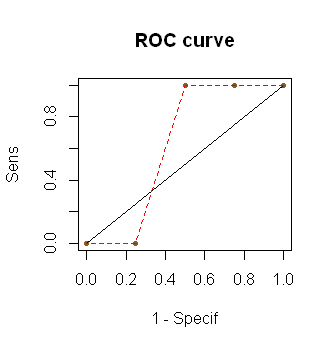In this particular example, then, the third cutoff or threshold point $$\color{red}{0.115}$$, associated with a probability of $$0.16395$$ would result in the highest combination of sensitivity and specificity.

If the probabilities of “success”" (outcome 1 or, most commonly, “disease”) predicted by the logistic regression order the values perfectly along the actual presence of “success”, we could end up with a system like: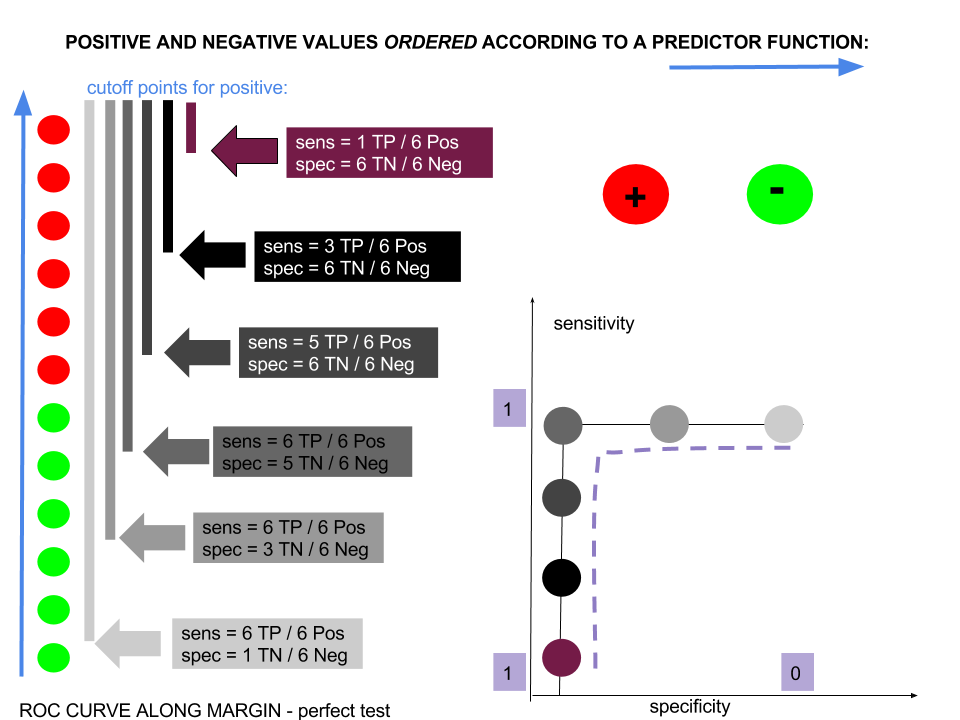In contradistinction, in a really bad test the predicted probabilities would bear no relation to the actual presence of the outcome, and the ROC curve would form a perfect diagonal: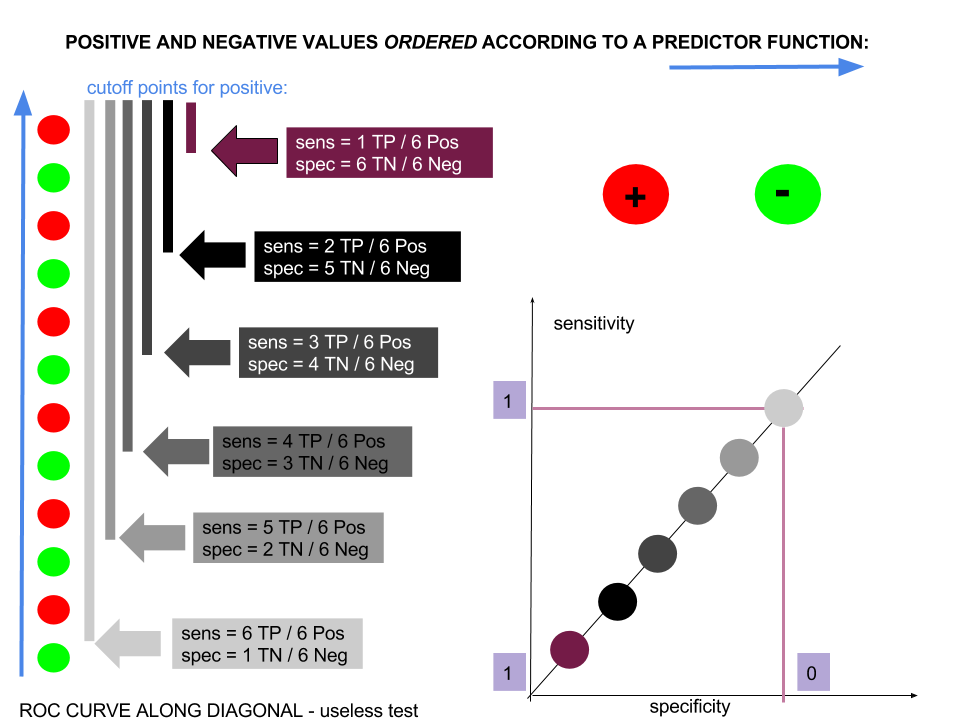In general, though, the shape of an ROC curve will tend to be something like: